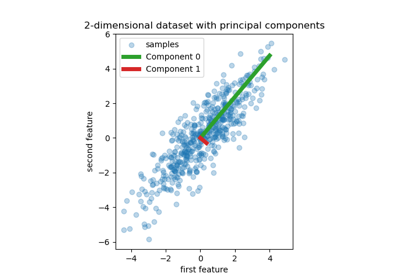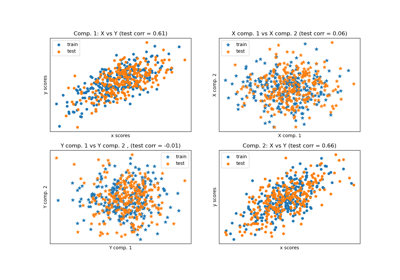# sklearn.cross_decomposition.PLSRegression¶

class sklearn.cross_decomposition.PLSRegression(n_components=2, *, scale=True, max_iter=500, tol=1e-06, copy=True)[source]

PLS regression

PLSRegression is also known as PLS2 or PLS1, depending on the number of targets.

Read more in the User Guide.

New in version 0.8.

Parameters
n_componentsint, default=2

Number of components to keep. Should be in [1, min(n_samples, n_features, n_targets)].

scalebool, default=True

Whether to scale X and Y.

algorithm{‘nipals’, ‘svd’}, default=’nipals’

The algorithm used to estimate the first singular vectors of the cross-covariance matrix. ‘nipals’ uses the power method while ‘svd’ will compute the whole SVD.

max_iterint, default=500

The maximum number of iterations of the power method when algorithm='nipals'. Ignored otherwise.

tolfloat, default=1e-06

The tolerance used as convergence criteria in the power method: the algorithm stops whenever the squared norm of u_i - u_{i-1} is less than tol, where u corresponds to the left singular vector.

copybool, default=True

Whether to copy X and Y in fit before applying centering, and potentially scaling. If False, these operations will be done inplace, modifying both arrays.

Attributes
x_weights_ndarray of shape (n_features, n_components)

The left singular vectors of the cross-covariance matrices of each iteration.

y_weights_ndarray of shape (n_targets, n_components)

The right singular vectors of the cross-covariance matrices of each iteration.

The loadings of X.

The loadings of Y.

x_scores_ndarray of shape (n_samples, n_components)

The transformed training samples.

y_scores_ndarray of shape (n_samples, n_components)

The transformed training targets.

x_rotations_ndarray of shape (n_features, n_components)

The projection matrix used to transform X.

y_rotations_ndarray of shape (n_features, n_components)

The projection matrix used to transform Y.

coef_ndarray of shape (n_features, n_targets)

The coefficients of the linear model such that Y is approximated as Y = X @ coef_.

n_iter_list of shape (n_components,)

Number of iterations of the power method, for each component. Empty if algorithm='svd'.

Examples

>>> from sklearn.cross_decomposition import PLSRegression
>>> X = [[0., 0., 1.], [1.,0.,0.], [2.,2.,2.], [2.,5.,4.]]
>>> Y = [[0.1, -0.2], [0.9, 1.1], [6.2, 5.9], [11.9, 12.3]]
>>> pls2 = PLSRegression(n_components=2)
>>> pls2.fit(X, Y)
PLSRegression()
>>> Y_pred = pls2.predict(X)


Methods

 fit(X, Y) Fit model to data. fit_transform(X[, y]) Learn and apply the dimension reduction on the train data. get_params([deep]) Get parameters for this estimator. Transform data back to its original space. predict(X[, copy]) Predict targets of given samples. score(X, y[, sample_weight]) Return the coefficient of determination $$R^2$$ of the prediction. set_params(**params) Set the parameters of this estimator. transform(X[, Y, copy]) Apply the dimension reduction.
fit(X, Y)[source]

Fit model to data.

Parameters
Xarray-like of shape (n_samples, n_features)

Training vectors, where n_samples is the number of samples and n_features is the number of predictors.

Yarray-like of shape (n_samples,) or (n_samples, n_targets)

Target vectors, where n_samples is the number of samples and n_targets is the number of response variables.

fit_transform(X, y=None)[source]

Learn and apply the dimension reduction on the train data.

Parameters
Xarray-like of shape (n_samples, n_features)

Training vectors, where n_samples is the number of samples and n_features is the number of predictors.

yarray-like of shape (n_samples, n_targets), default=None

Target vectors, where n_samples is the number of samples and n_targets is the number of response variables.

Returns
x_scores if Y is not given, (x_scores, y_scores) otherwise.
get_params(deep=True)[source]

Get parameters for this estimator.

Parameters
deepbool, default=True

If True, will return the parameters for this estimator and contained subobjects that are estimators.

Returns
paramsdict

Parameter names mapped to their values.

inverse_transform(X)[source]

Transform data back to its original space.

Parameters
Xarray-like of shape (n_samples, n_components)

New data, where n_samples is the number of samples and n_components is the number of pls components.

Returns
x_reconstructedarray-like of shape (n_samples, n_features)

Notes

This transformation will only be exact if n_components=n_features.

predict(X, copy=True)[source]

Predict targets of given samples.

Parameters
Xarray-like of shape (n_samples, n_features)

Samples.

copybool, default=True

Whether to copy X and Y, or perform in-place normalization.

Notes

This call requires the estimation of a matrix of shape (n_features, n_targets), which may be an issue in high dimensional space.

score(X, y, sample_weight=None)[source]

Return the coefficient of determination $$R^2$$ of the prediction.

The coefficient $$R^2$$ is defined as $$(1 - \frac{u}{v})$$, where $$u$$ is the residual sum of squares ((y_true - y_pred) ** 2).sum() and $$v$$ is the total sum of squares ((y_true - y_true.mean()) ** 2).sum(). The best possible score is 1.0 and it can be negative (because the model can be arbitrarily worse). A constant model that always predicts the expected value of y, disregarding the input features, would get a $$R^2$$ score of 0.0.

Parameters
Xarray-like of shape (n_samples, n_features)

Test samples. For some estimators this may be a precomputed kernel matrix or a list of generic objects instead with shape (n_samples, n_samples_fitted), where n_samples_fitted is the number of samples used in the fitting for the estimator.

yarray-like of shape (n_samples,) or (n_samples, n_outputs)

True values for X.

sample_weightarray-like of shape (n_samples,), default=None

Sample weights.

Returns
scorefloat

$$R^2$$ of self.predict(X) wrt. y.

Notes

The $$R^2$$ score used when calling score on a regressor uses multioutput='uniform_average' from version 0.23 to keep consistent with default value of r2_score. This influences the score method of all the multioutput regressors (except for MultiOutputRegressor).

set_params(**params)[source]

Set the parameters of this estimator.

The method works on simple estimators as well as on nested objects (such as Pipeline). The latter have parameters of the form <component>__<parameter> so that it’s possible to update each component of a nested object.

Parameters
**paramsdict

Estimator parameters.

Returns
selfestimator instance

Estimator instance.

transform(X, Y=None, copy=True)[source]

Apply the dimension reduction.

Parameters
Xarray-like of shape (n_samples, n_features)

Samples to transform.

Yarray-like of shape (n_samples, n_targets), default=None

Target vectors.

copybool, default=True

Whether to copy X and Y, or perform in-place normalization.

Returns
x_scores if Y is not given, (x_scores, y_scores) otherwise.

## Examples using sklearn.cross_decomposition.PLSRegression¶